Next: The Zeldovich approximation Up: Describing the inhomogeneities of Previous: Describing the inhomogeneities of

## The linear approximation

A particularly simple case occurs when dealing with very small inhomogeneities. This is the case either at very early epochs or at the present time, when considering sufficiently large scales, so that the variance of the galaxy number counts is much less than unity. In this regime,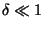and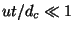,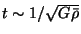being the expansion time-scale and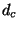the characteristic coherence length of the fluctuation field. This enables us to neglect in eqs.(12) all the non-linear terms, to get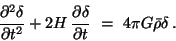(13)

Note that the linear approximation amounts to assuming no phase coupling, so that fluctuation modes at different wavelengths evolve independent of each other.

In the case of a flat Universe with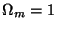, no cosmological constant, and matter dominated expansion, the Friedmann equations give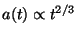, and eq.(13) has the general solution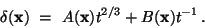(14)

This solution essentially says that perturbation evolution is given by the superposition of a growing and a decaying mode, that becomes rapidly negligible as expansion goes on. It is interesting to note that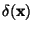grows at the same rate as the scale factor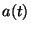. This is nothing but the consequence of the similarity between the gravitational collapse time-scale,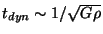, and the expansion time-scale,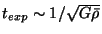, that occurs in thecase. This result is valid both for a flat Universe and for an open Universe (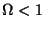) at sufficiently early times, when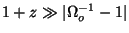and the spatial curvature can be neglected. However, in the last case, at later times the cosmic expansion rate increases, so that the fluctuation growing stops and their amplitudes are frozen. Thus, in order to allow observed structures to be formed, a not too low density parameter is required.

As the typical fluctuation amplitude approaches unity at a given scale, phase coupling terms in eqs.(12) start playing a role and the linear approximation breaks down. In this case, a rigorous treatment for inhomogeneity evolution has not yet been derived, although different prescriptions have been developed to get hints about non-linear aspects of gravitational clustering.

As a direct approach, one can perturbatively expand eqs.() in terms of increasing order in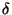and try to solve the system order by order. While linear equations preserve initial Gaussianity, the inclusion of the lowest-order non-linear terms gives rise to a non vanishing skewness of the fluctuation probability distribution , that is the signature of non-Gaussian statistics. This approximation is expected to hold in the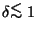regime, while in the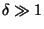regime the convergence of the perturbative expansion series is no longer guaranteed.Next: The Zeldovich approximation Up: Describing the inhomogeneities of Previous: Describing the inhomogeneities of
Waleska Aldana Segura 2001-01-16